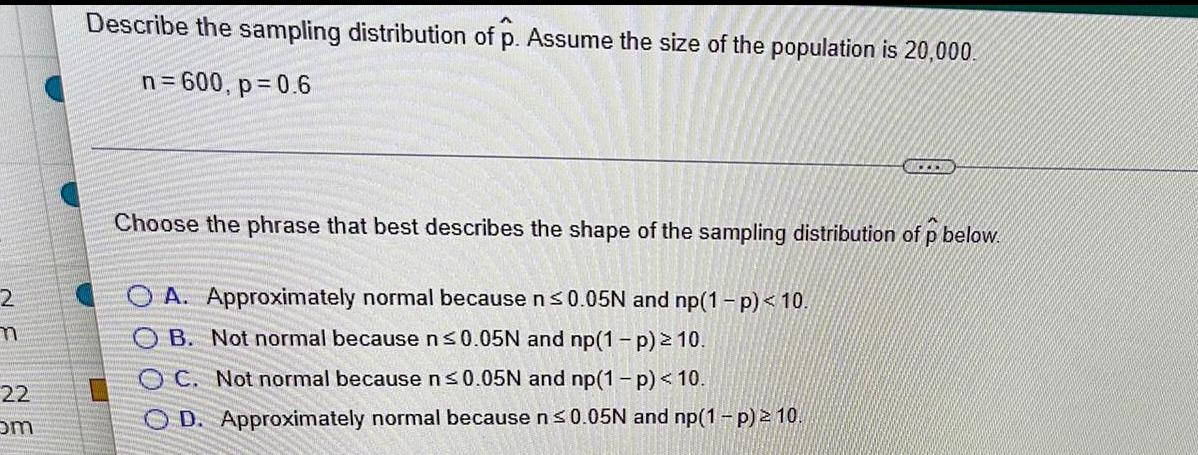Question:

# 2 m 22 om Describe the sampling distribution of p. Assume the size of the population is 20,000. n = 600, p=0.6 ... Choose the phrase that best describes the shape of the sampling distribution of p below. OA. Approximately normal because n ≤0.05N and

Last updated: 8/15/2022Describe the sampling distribution of p. Assume the size of the population is 20,000. n = 600, p=0.6 Choose the phrase that best describes the shape of the sampling distribution of p below. A. Approximately normal because n≤0.05N and np(1-p)<10. B. Not normal because n≤0.05N and np(1-p)≥10. C. Not normal because n≤0.05N and np(1-p)<10. D. Approximately normal because n≤0.05N and np(1-p)≥10.